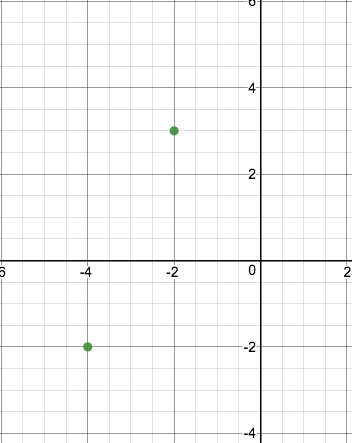# LINR 1 | Lesson 4 | Try This! (Write Equations Using $$\boldsymbol{y=mx+b}$$)

## Write Equations Using $$y=mx+b$$

Consider the graph below containing the points $$(-4, -2)$$ and $$(-2, 3)$$.1. What is the slope of the line containing these two points?

1. If we use the slope to count the rise and run, what are the coordinates of the $$y$$-intercept?

1. Write the equation of this line in slope-intercept form.

Remember: We can determine the slope between any two points using the slope-formula.  Given Point A: $$(-4, -2)$$ and Point B: $$(-2, 3)$$ we calculate the slope as:

$slope=\dfrac{3-(-2)}{-2-(-4)}=\dfrac{3+2}{-2+4}=\dfrac{5}{2}$

Additionally, we can use the slope-intercept form of the line to determine the $$y$$-intercept, as shown below.  Finding the $$y$$-intercept in this manner is useful when counting using the slope is impractical or cumbersome.

By plugging in the slope for $$m$$, and one point on the line for $$x$$ and $$y$$, we can find the $$y$$-intercept.

\begin{align} y&=mx+b \\\\ 2&=\dfrac{5}{2}(-4)+b \\\\ 2&=-10+b \\\\ 8&=b\end{align}

Therefore the equation of the line is: $$y=\dfrac{5}{2}x+8$$.

1. Use this method to find the equation of the line between the points $$( -2, -1)$$ and $$(3, 2)$$.

Check solutions here.